Circle

Chapter 11 Class 11 Conic Sections
Concept wise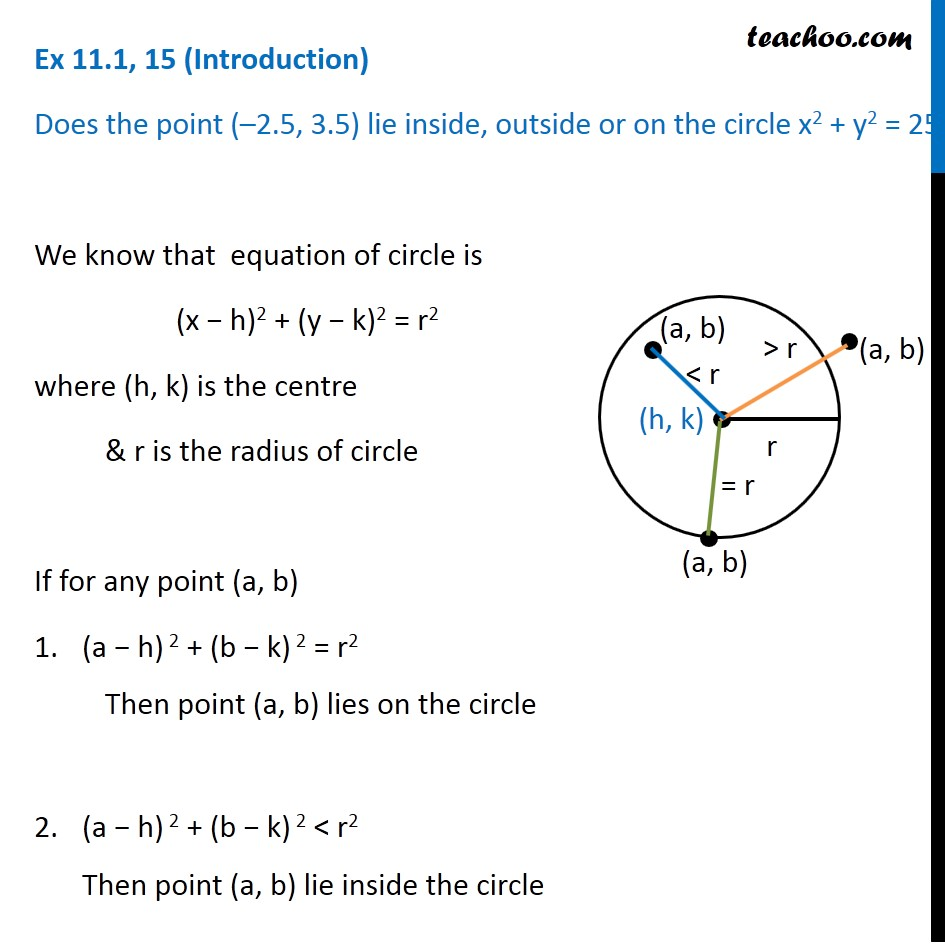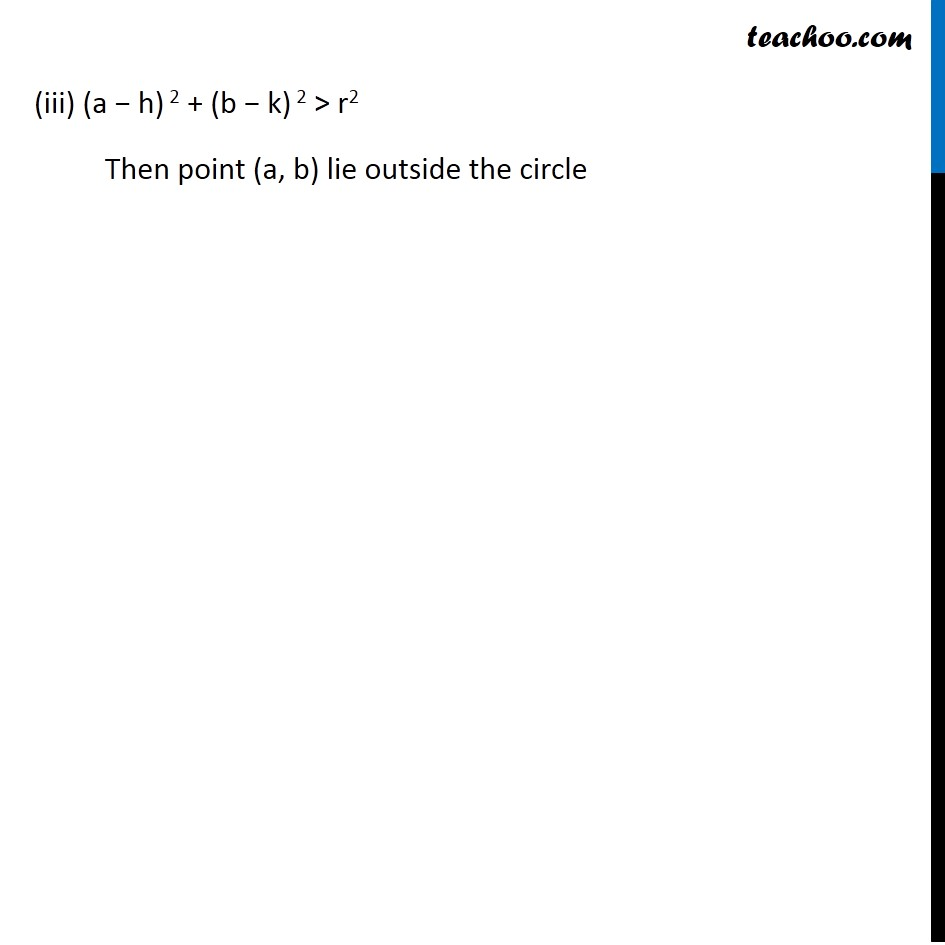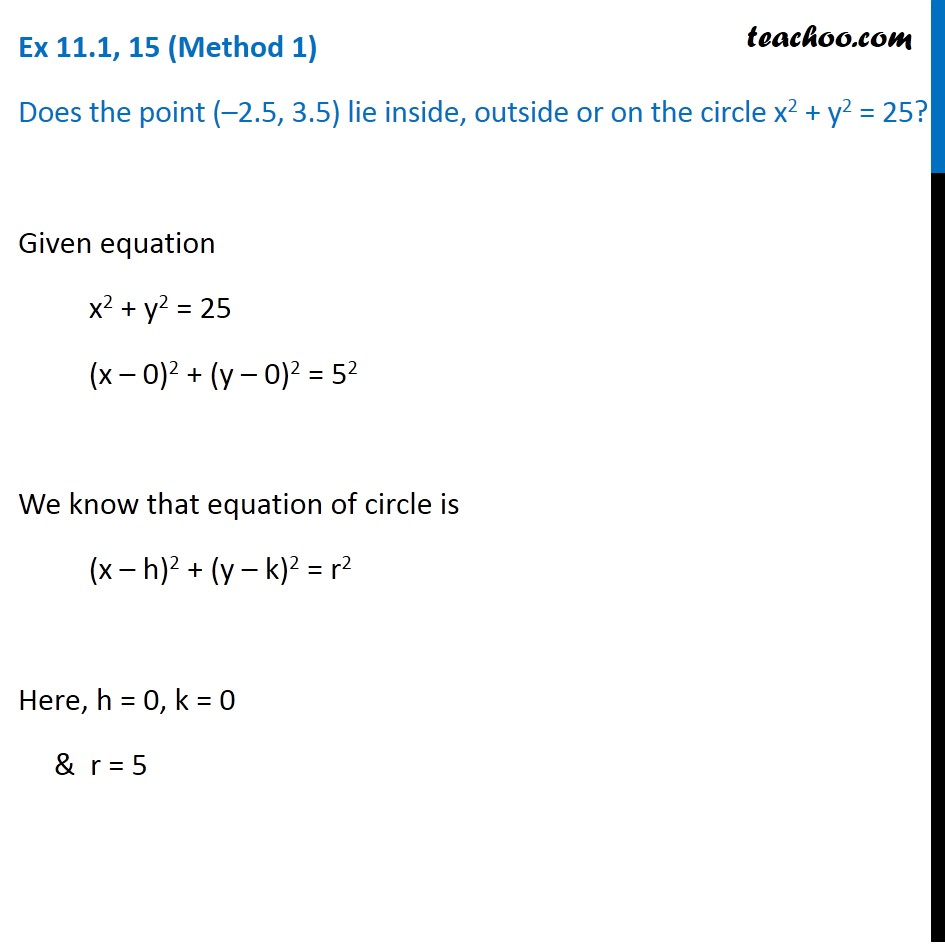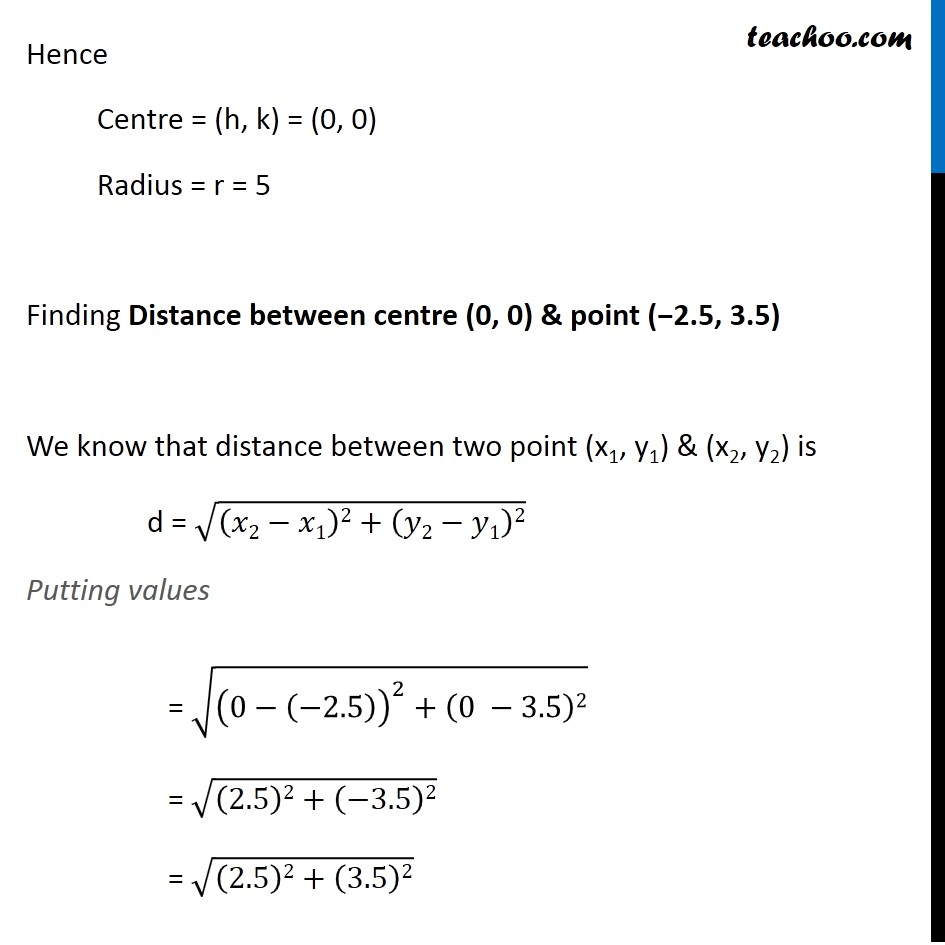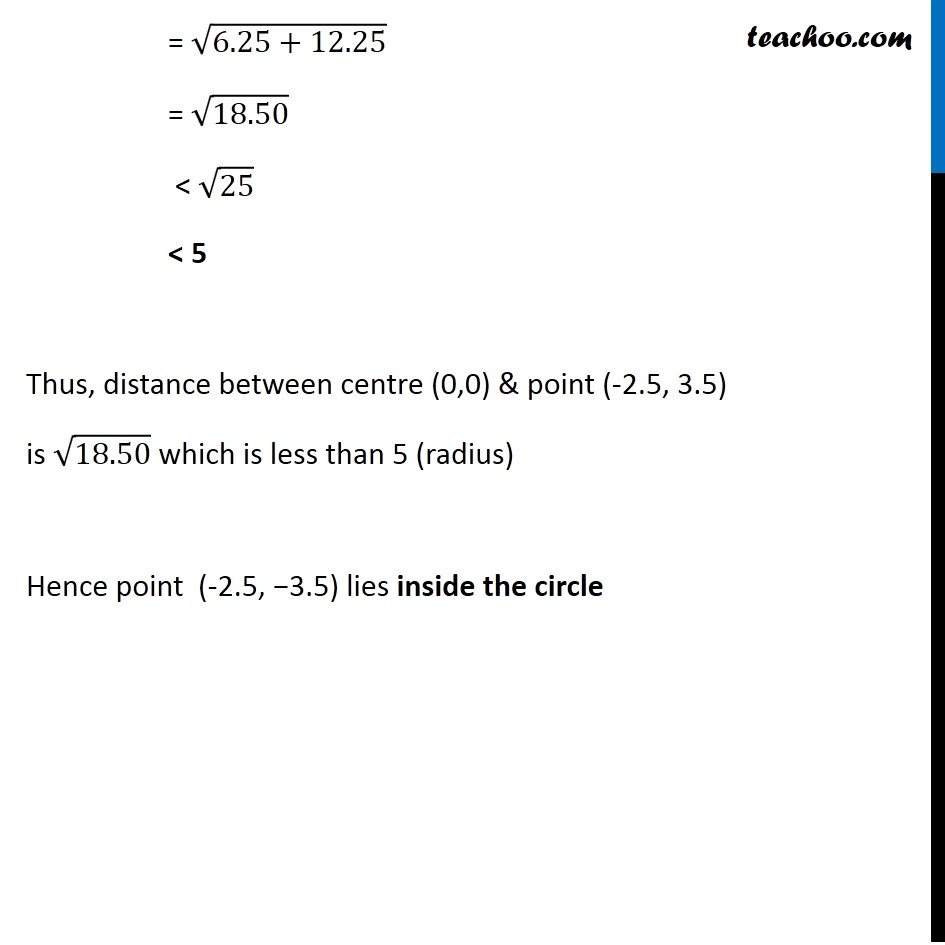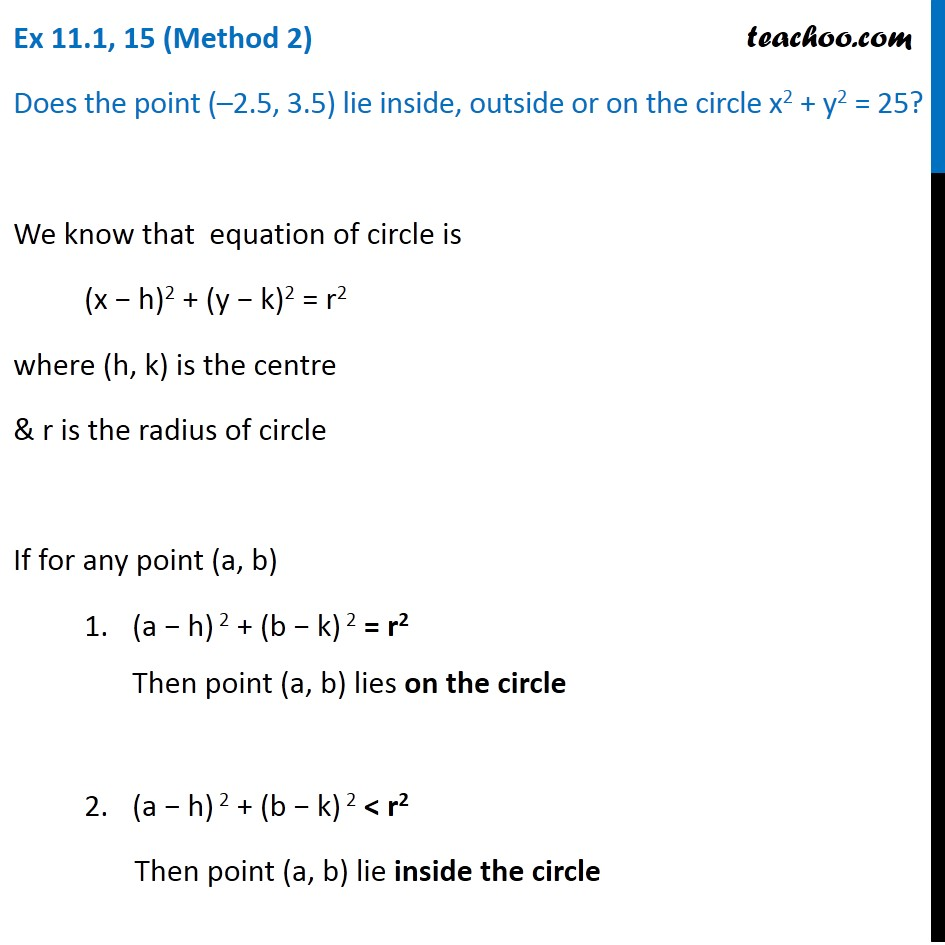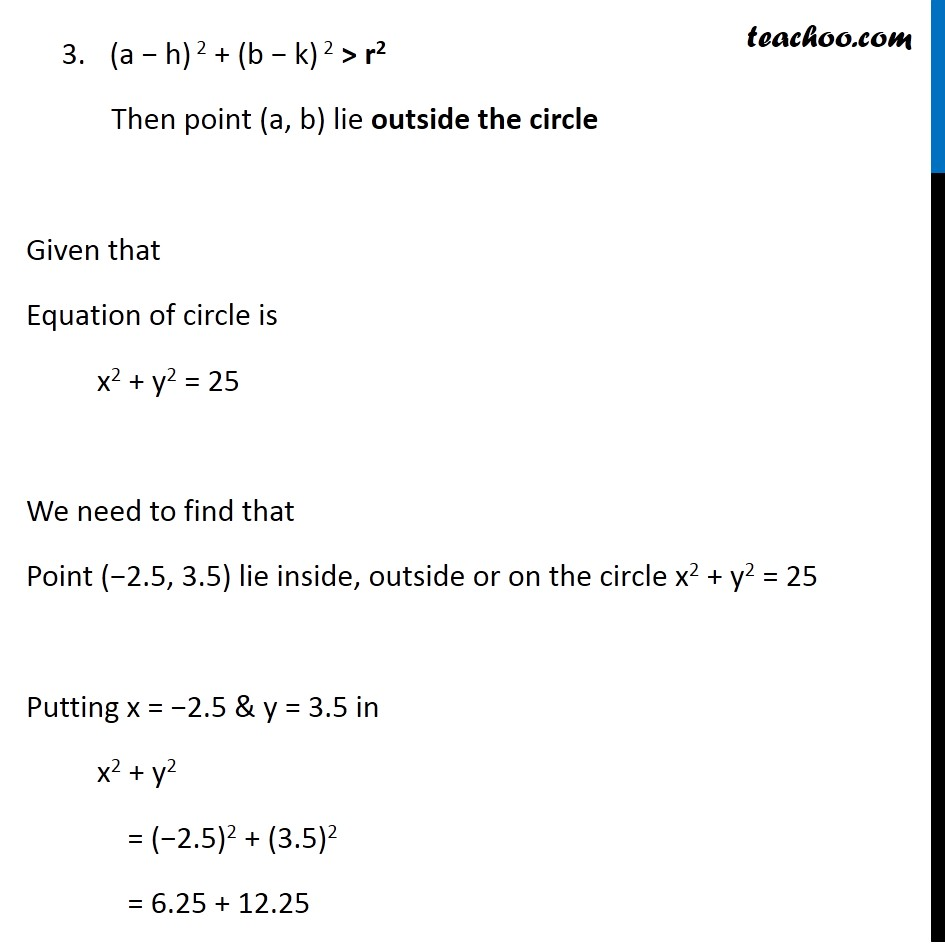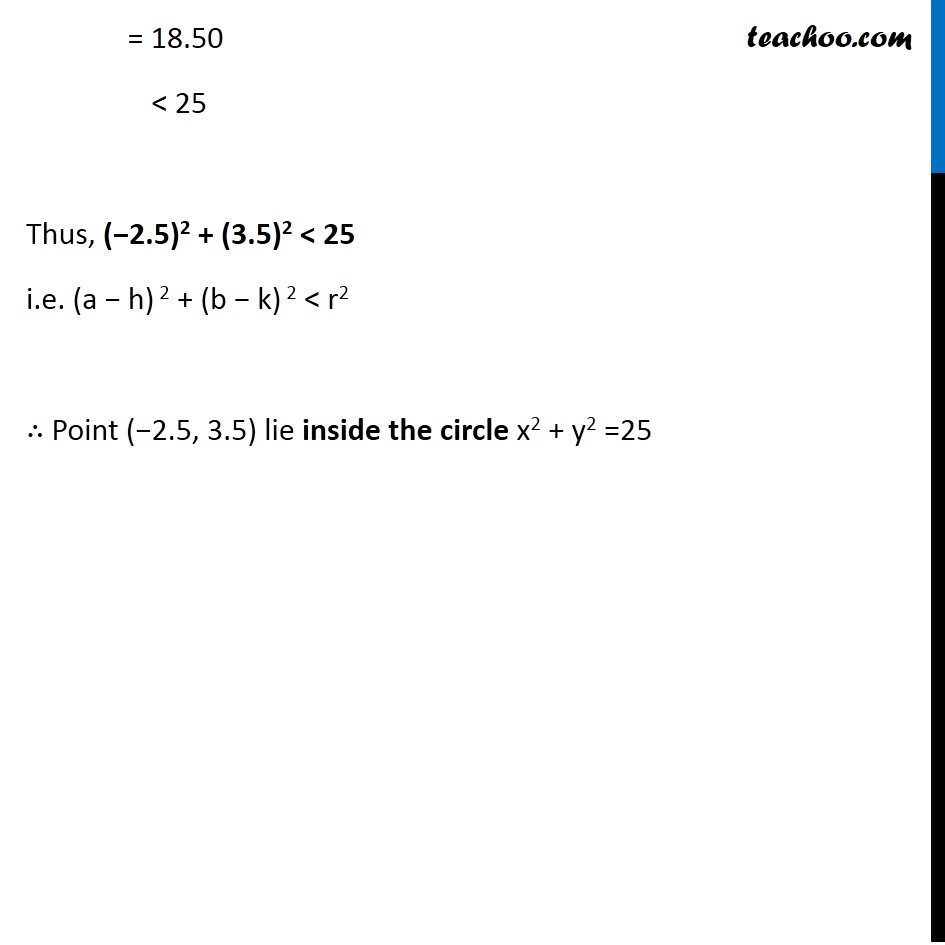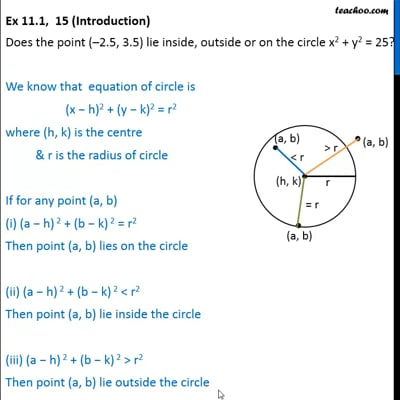This video is only available for Teachoo black users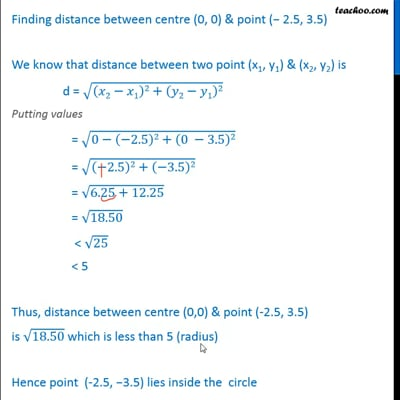This video is only available for Teachoo black users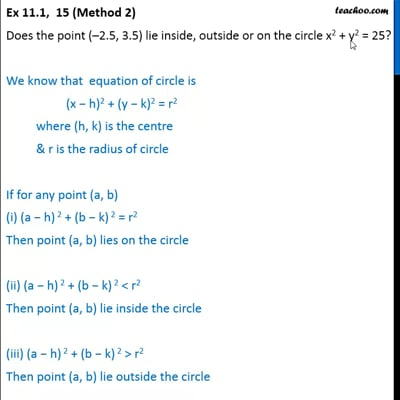This video is only available for Teachoo black users

Introducing your new favourite teacher - Teachoo Black, at only ₹83 per month

### Transcript

Ex 11.1, 15 (Introduction) Does the point (–2.5, 3.5) lie inside, outside or on the circle x2 + y2 = 25? We know that equation of circle is (x − h)2 + (y − k)2 = r2 where (h, k) is the centre & r is the radius of circle If for any point (a, b) (a − h) 2 + (b − k) 2 = r2 Then point (a, b) lies on the circle (a − h) 2 + (b − k) 2 < r2 Then point (a, b) lie inside the circle (iii) (a − h) 2 + (b − k) 2 > r2 Then point (a, b) lie outside the circle Ex 11.1, 15 (Method 1) Does the point (–2.5, 3.5) lie inside, outside or on the circle x2 + y2 = 25? Given equation x2 + y2 = 25 (x – 0)2 + (y – 0)2 = 52 We know that equation of circle is (x – h)2 + (y – k)2 = r2 Here, h = 0, k = 0 & r = 5 Hence Centre = (h, k) = (0, 0) Radius = r = 5 Finding Distance between centre (0, 0) & point (−2.5, 3.5) We know that distance between two point (x1, y1) & (x2, y2) is d = √((𝑥2−𝑥1)2+(𝑦2−𝑦1)2) Putting values = √((0−(−2.5))^2+(0 −3.5)2) = √((2.5)2+(−3.5)2) = √((2.5)2+(3.5)2) = √(6.25+12.25) = √18.50 < √25 < 5 Thus, distance between centre (0,0) & point (-2.5, 3.5) is √18.50 which is less than 5 (radius) Hence point (-2.5, −3.5) lies inside the circle Ex 11.1, 15 (Method 2) Does the point (–2.5, 3.5) lie inside, outside or on the circle x2 + y2 = 25? We know that equation of circle is (x − h)2 + (y − k)2 = r2 where (h, k) is the centre & r is the radius of circle If for any point (a, b) (a − h) 2 + (b − k) 2 = r2 Then point (a, b) lies on the circle (a − h) 2 + (b − k) 2 < r2 Then point (a, b) lie inside the circle (a − h) 2 + (b − k) 2 > r2 Then point (a, b) lie outside the circle Given that Equation of circle is x2 + y2 = 25 We need to find that Point (−2.5, 3.5) lie inside, outside or on the circle x2 + y2 = 25 Putting x = −2.5 & y = 3.5 in x2 + y2 = (−2.5)2 + (3.5)2 = 6.25 + 12.25 = 18.50 < 25 Thus, (−2.5)2 + (3.5)2 < 25 i.e. (a − h) 2 + (b − k) 2 < r2 ∴ Point (−2.5, 3.5) lie inside the circle x2 + y2 =25